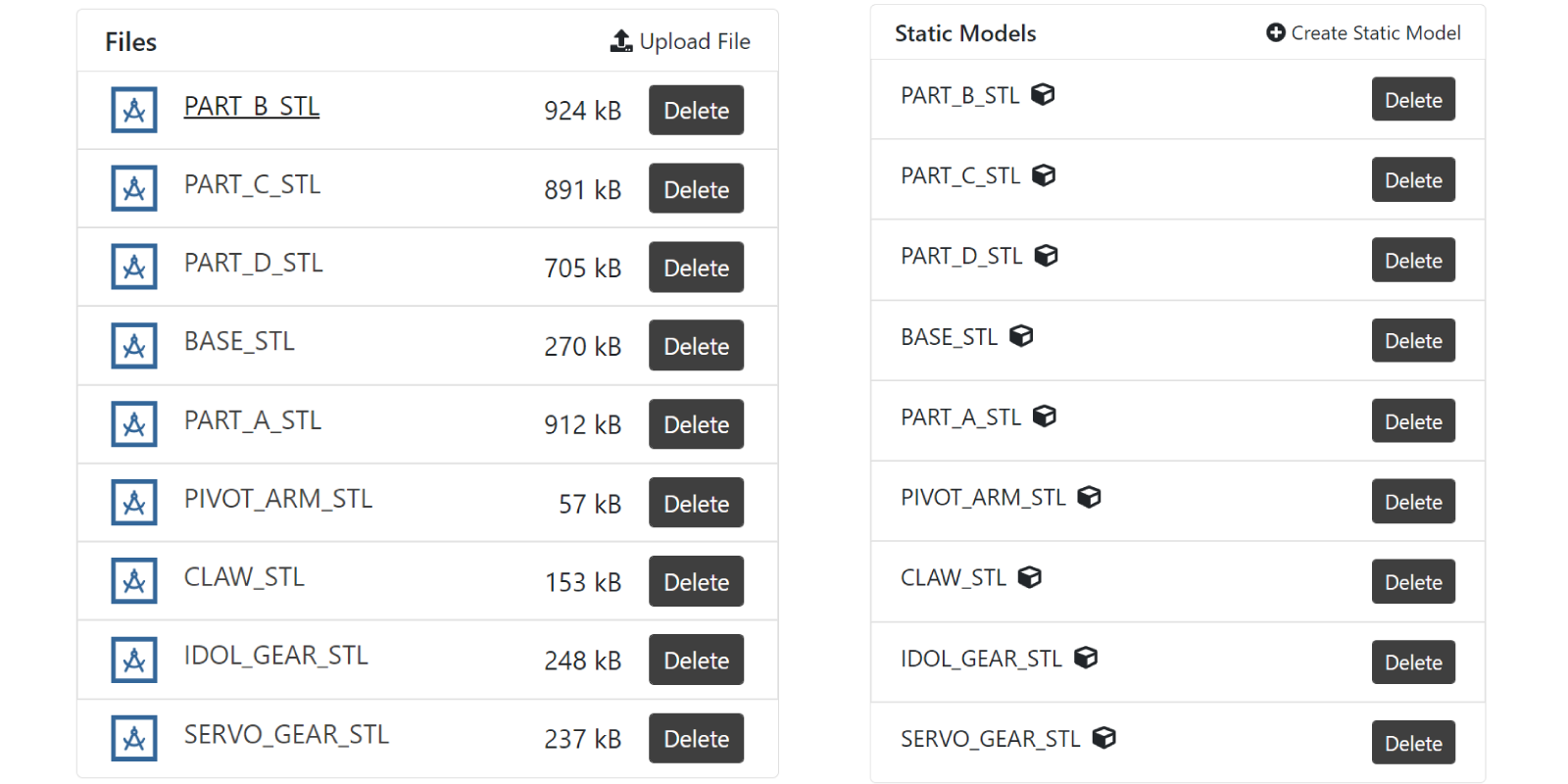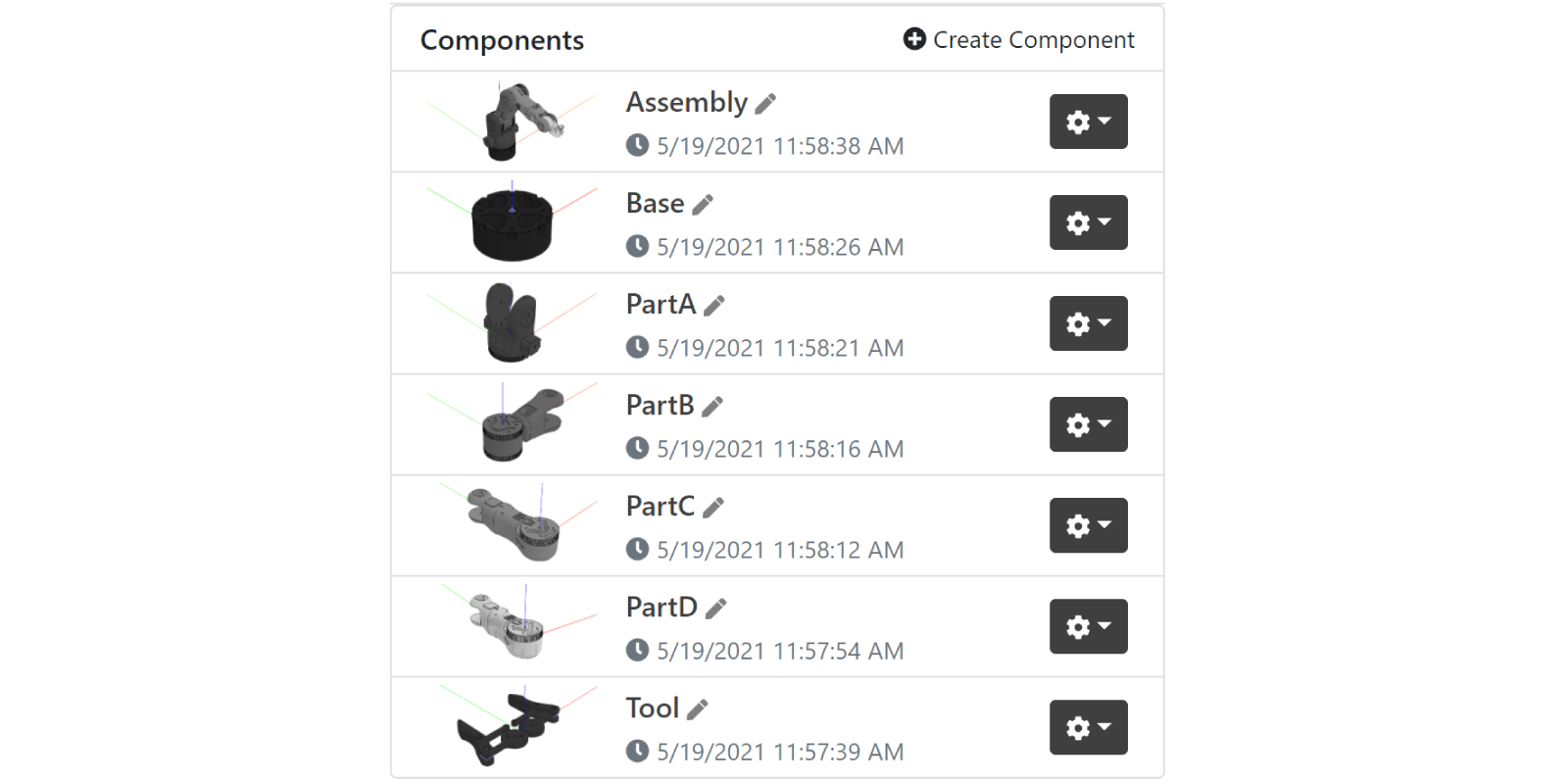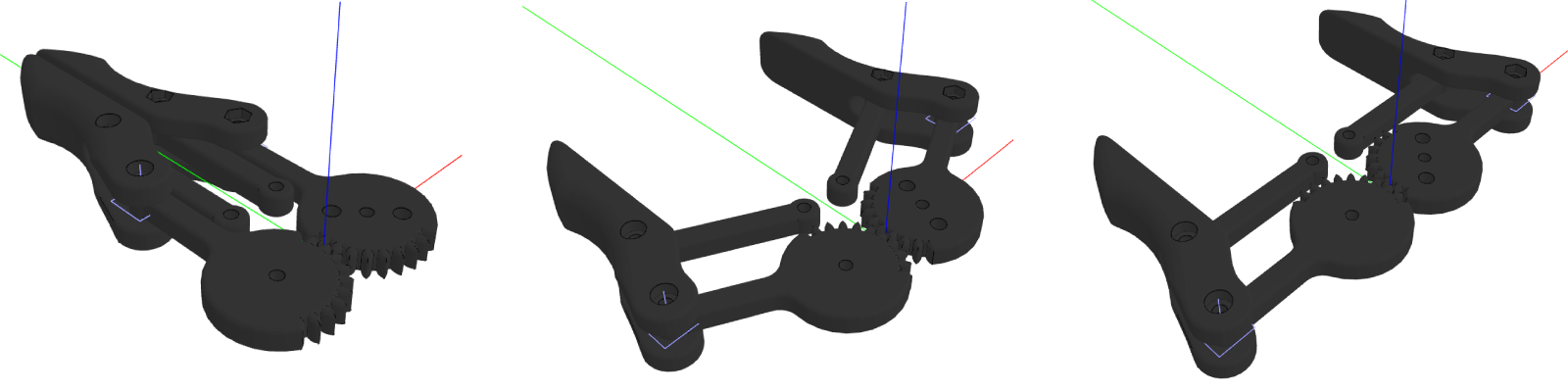# How To Use Connectors

In DynaMaker it is very easy to add components at specific points of other components. By using connectors as a bundle of position and rotation, subcomponents can be added through the component handler in a simpler way.

To explain how they work, we take a robot arm as the product example. Each part or component of the robot will contain a list of connectors. This robot will be modelled from an open-source robot arm and put in an app by following these steps:

### A. Prepare Components​

To make it simpler for this example, we have prepared and assembled the parts accordingly so the STL files are lighter and ready for use with connectors, but feel free to use the full model instead with all the lids and screws if you wish. A component will be created for each main part of the robot arm. Then, connectors will be added accordingly and finally, the assembly will know how to populate those connectors with the respective subcomponents. Let's go step by step:

• Download all the simpler STL files together with the official manual here.
• Upload them to the DynaMaker project and create a static model for each:• Create a component for each part together with an Assembly component. You can bundle the claw, gears and pivot arms into Tool, so the final result would look something like this:Once we have the components ready, let's add the connectors for each one of them.

### B. Subcomponent Connectors​

To cache the models properly, they need to be created outside `generateGeometry()`, then they can be added to the geometry with the position and rotation desired.

• In GEOM3D, create the model from the static model as:
``export const baseModel = ASSETS.STATIC_MODELS.BASE_STL``
• In COMPONENT, add the model to the geometry in `generateGeometry()` with a color, like:
``generateGeometry() {  const geometryGroup = new SKYCAD.GeometryGroup()  geometryGroup.addGeometry(GEOM3D.baseModel, {    materials: [new SKYCAD.Material({ color: 0x222222 })],  })  return geometryGroup}``
• Add a connector for the following part (Part A in this case) within the `class` in the standard function `getConnectors()`. Also, add a `tag` so it's easier to retrieve later in the component Assembly:
``getConnectors() {  return [    new SKYCAD.Connector3D({ position: new SKYMATH.Vector3D(0, 0, 65), tags: ['part-a'] }),  ]}``

See that using the function `getConnectors()`, which returns a list of connectors, will result in visualizing the connectors in the current Component Editor as a small blue-ish coordinate system.Repeat these 3 steps for parts A through D (you can expand the sections below) and leave the Tool for the following section.

Show Part A logic:
``// GEOM / GEOM3Dexport const partAModel = ASSETS.STATIC_MODELS.PART_A_STL// COMP / ComponentgetConnectors() {  return [    new SKYCAD.Connector3D({      position: new SKYMATH.Vector3D(0, 0, 165),      rotation: new SKYMATH.Vector3D(-Math.PI / 2, 0, -Math.PI / 2),      tags: ['part-b']    }),  ]}generateGeometry() {  const geometryGroup = new SKYCAD.GeometryGroup()  geometryGroup.addGeometry(GEOM3D.partAModel, {    materials: [new SKYCAD.Material({ color: 0X444444 })],    rotation: new SKYMATH.Vector3D(0, 0, Math.PI / 2),  })  return geometryGroup}``
Show Part B logic:
``// GEOM / GEOM3Dexport const partBModel = ASSETS.STATIC_MODELS.PART_B_STL// COMP / ComponentgetConnectors() {  return [    new SKYCAD.Connector3D({      position: new SKYMATH.Vector3D(220, 0, 0),      rotation: new SKYMATH.Vector3D(0, 0, -Math.PI / 2),      tags: ['part-c']    }),  ]}generateGeometry() {  const geometryGroup = new SKYCAD.GeometryGroup()  geometryGroup.addGeometry(GEOM3D.partBModel, {    materials: [new SKYCAD.Material({ color: 0X666666 })],    rotation: new SKYMATH.Vector3D(0, 0, -Math.PI / 2)  })  return geometryGroup}``
Show Part C logic:
``// GEOM / GEOM3Dexport const partCModel = ASSETS.STATIC_MODELS.PART_C_STL// COMP / ComponentgetConnectors() {  return [    new SKYCAD.Connector3D({      position: new SKYMATH.Vector3D(0, 222.5, 0),      rotation: new SKYMATH.Vector3D(Math.PI , 0, Math.PI),      tags: ['part-d']    }),  ]}generateGeometry() {  const geometryGroup = new SKYCAD.GeometryGroup()  geometryGroup.addGeometry(GEOM3D.partCModel, {    materials: [new SKYCAD.Material({ color: 0X888888 })],    position: new SKYMATH.Vector3D(-35, 127.5, 0),    rotation: new SKYMATH.Vector3D(0, 0, - Math.PI / 2),  })  return geometryGroup}``
Show Part D logic:
``// GEOM / GEOM3Dexport const partDModel = ASSETS.STATIC_MODELS.PART_D_STL// COMP / ComponentgetConnectors() {  return [    new SKYCAD.Connector3D({      position: new SKYMATH.Vector3D(0, 65, 0),      rotation: new SKYMATH.Vector3D(0, -Math.PI / 2, 0),      tags: ['tool']    }),  ]}generateGeometry() {  const geometryGroup = new SKYCAD.GeometryGroup()  geometryGroup.addGeometry(GEOM3D.partDModel, {    materials: [new SKYCAD.Material({ color: 0XBBBBBB, opacity: 0.95 })], // some opacity will help to see how the gears move inside  })  return geometryGroup}``Other values for positions and rotations of the models and connectors may be valid as well depending on how they are connected with the parameters later and their default values.

#### Tool logic​

The tool of the robot arm consists of 4 different parts: the claws, the left and right gear that are connected to, together with the pivot arms that strengthen the movement. We will include a property `openingPercentage` that tells how much the tool is open so that the angle with which the parts are rotated will be easier to calculate. For this:

• In CONSTANTS update `Properties`:
``export interface Properties {  openingPercentage: number}``
• In COMPONENT update the component properties:
``this.properties = {  openingPercentage: 0.7,}``
• In GEOM3D create the 4 models from the static assets:
``export const clawModel = ASSETS.STATIC_MODELS.CLAW_STLexport const pivotArmModel = ASSETS.STATIC_MODELS.PIVOT_ARM_STLexport const idolGearModel = ASSETS.STATIC_MODELS.IDOL_GEAR_STLexport const servoGearModel = ASSETS.STATIC_MODELS.SERVO_GEAR_STL``
• In COMPONENT within the `class`, create `getAngle()` that calculates the angle for the rotation of the parts, based on `openingPercentage` and add the models to the geometry in `generateGeometry()` with the help of `getConnectors()` to add the claws so they don't rotate with `openingPercentage`.
``getAngle() {  const { openingPercentage } = this.properties  return (1 - openingPercentage) * 90 * Math.PI / 180}getConnectors() {  const angle = this.getAngle()  return [    new SKYCAD.Connector3D({      position: new SKYMATH.Vector3D(-15 - 40 * Math.cos(angle), + 40 * Math.sin(angle), 0),      rotation: new SKYMATH.Vector3D(0, 0, 0),      tags: ['left-claw']    }),    new SKYCAD.Connector3D({      position: new SKYMATH.Vector3D(15 + 40 * Math.cos(angle), + 40 * Math.sin(angle), 0),      rotation: new SKYMATH.Vector3D(0, Math.PI, 0),      tags: ['right-claw']    }),  ]}generateGeometry() {  const angle = this.getAngle()  const geometryGroup = new SKYCAD.GeometryGroup()  // Gears  geometryGroup.addGeometry(GEOM3D.servoGearModel, {    materials: [new SKYCAD.Material({ color: 0X333333 })],    position: new SKYMATH.Vector3D(14, 0, 0),    rotation: new SKYMATH.Vector3D(0, 0, angle),  })  geometryGroup.addGeometry(GEOM3D.idolGearModel, {    materials: [new SKYCAD.Material({ color: 0X333333 })],    position: new SKYMATH.Vector3D(-14, 0, 0),    rotation: new SKYMATH.Vector3D(0, 0, -angle),  })  // Pivot arms  geometryGroup.addGeometry(GEOM3D.pivotArmModel, {    materials: [new SKYCAD.Material({ color: 0X333333 })],    position: new SKYMATH.Vector3D(7, 20, -3),    rotation: new SKYMATH.Vector3D(0, 0, angle),  })  geometryGroup.addGeometry(GEOM3D.pivotArmModel, {    materials: [new SKYCAD.Material({ color: 0X333333 })],    position: new SKYMATH.Vector3D(-7, 20, -3),    rotation: new SKYMATH.Vector3D(0, 0, Math.PI - angle),  })  // Claws  const clawsConnectors = this.getConnectors()  const leftConnector = clawsConnectors.filter(connector => connector.hasTag('left-claw'))  const rightConnector = clawsConnectors.filter(connector => connector.hasTag('right-claw'))  geometryGroup.addGeometry(GEOM3D.clawModel, {    materials: [new SKYCAD.Material({ color: 0X333333 })],    connector: leftConnector  })  geometryGroup.addGeometry(GEOM3D.clawModel, {    materials: [new SKYCAD.Material({ color: 0X333333 })],    connector: rightConnector  })  return geometryGroup}``

As a result of the above, you should have a model that looks like the following for `openingPercentage = 0`, `openingPercentage = 0.7` and `openingPercentage = 1` respectively:Notice that the pivot arms and the gears rotate with the angle, but not the claws since they are placed in the connectors with the tags `'left-claw'` and `'right-claw'`.

Great! Now that we have all the components, it's time to assemble them. To add the subcomponents, we will follow a similar process as we did in the Tool component, i.e. taking the connectors of each part to add its following.

## C. Assembly​

Let's start with the properties. We will add a property with the rotation for each part and an extra one to connect with the mentioned `openingPercentage` tool property. Then, we will update the parameters, so we get the right configurator in the app. And finally, add the components as subcomponents to the assembly, so that the geometry is generated from all of them.

#### Properties​

• In CONSTANTS update `Properties`:
``export interface Properties {  partARotation: number  partBRotation: number  partCRotation: number  partDRotation: number  openingPercentage: number}``
• In COMPONENT update the component properties and call `update()` for later within the `constructor`:
`` constructor() {  super()  this.properties = {    partARotation: 270 * Math.PI / 180,    partBRotation: 30 * Math.PI / 180,    partCRotation: 75 * Math.PI / 180,    partDRotation: 0 * Math.PI / 180,    openingPercentage: 1,  }  this.update() // this will handle the logic for adding subcomponents later}``

#### Parameters​

Update the configurator so that it has 4 parameters (1 for each part rotation) and a 5th for the opening percentage of the gripper tool.

``export function generateConfigurator(component: COMPONENT.Component): SKYPARAM.Configurator {  const partARotationParameter = SKYPARAM.generateSlider(PARAMETER.PART_A_ROTATION, 'Part A rotation', {    defaultValue: Math.round(component.getProperty('partARotation') * 180 / Math.PI),    maxValue: 359,    minValue: 0,    stepSize: 1,    unit: '°',  })  const partBRotationParameter = SKYPARAM.generateSlider(PARAMETER.PART_B_ROTATION, 'Part B rotation', {    defaultValue: Math.round(component.getProperty('partBRotation') * 180 / Math.PI),    maxValue: 120,    minValue: -120,    stepSize: 1,    unit: '°',  })  const partCRotationParameter = SKYPARAM.generateSlider(PARAMETER.PART_C_ROTATION, 'Part C rotation', {    defaultValue: Math.round(component.getProperty('partCRotation') * 180 / Math.PI),    maxValue: 120,    minValue: -120,    stepSize: 1,    unit: '°',  })  const partDRotationParameter = SKYPARAM.generateSlider(PARAMETER.PART_D_ROTATION, 'Part D rotation', {    defaultValue: Math.round(component.getProperty('partDRotation') * 180 / Math.PI),    maxValue: 120,    minValue: -120,    stepSize: 1,    unit: '°',  })  const openingPercentageParameter = SKYPARAM.generateSlider(PARAMETER.OPENING_PERCENTAGE, 'Opening Percentage', {    defaultValue: Math.round(component.getProperty('openingPercentage') * 100),    maxValue: 100,    minValue: 0,    stepSize: 1,    unit: '%',  })  const configurableParameters = [    partARotationParameter,    partBRotationParameter,    partCRotationParameter,    partDRotationParameter,    openingPercentageParameter,  ]  const configurator = new SKYPARAM.Configurator(configurableParameters)  configurator.addUpdateCallback((validUserInput, values) => {    if (validUserInput) {      component.setProperties({        partARotation: values[PARAMETER.PART_A_ROTATION] * Math.PI / 180,        partBRotation: values[PARAMETER.PART_B_ROTATION] * Math.PI / 180,        partCRotation: values[PARAMETER.PART_C_ROTATION] * Math.PI / 180,        partDRotation: values[PARAMETER.PART_D_ROTATION] * Math.PI / 180,        openingPercentage: values[PARAMETER.OPENING_PERCENTAGE] / 100,      })    }  })  return configurator}``

Instead of having fixed max/min values, you can try to add rules to make sure the parts don't collide with each other at all possible positions. Remember also that the values presented in the parameter can differ from the actual value stored in the component. For example, the angles are presented in degrees, while in the component radians are used to be consistent.

Use the function `update()` as always to clear, create and add subcomponents into the component handler. Also, use the connectors from the component to add the following part. Don't forget to connect the Assembly properties with the subcomponents.

``update() {  const { partARotation, partBRotation, partCRotation, partDRotation, openingPercentage } = this.properties  this.componentHandler.clearAll()  // Base  const baseComponent = new BASE.Component()  this.componentHandler.add(baseComponent)  const baseConnectors = this.componentHandler.getConnectors(BASE.Component)  // Part A  const partAConnectors = baseConnectors.filter(connector => connector.hasTag('part-a'))  const partAComponent = new PARTA.Component()  partAConnectors.forEach(connector => {    this.componentHandler.add(partAComponent, { connector, rotation: new SKYMATH.Vector3D(0, 0, partARotation) })  })  // Part B  const partBConnectors = this.componentHandler.getConnectors(PARTA.Component).filter(connector => connector.hasTag('part-b'))  const partBComponent = new PARTB.Component()  partBConnectors.forEach(connector => {    this.componentHandler.add(partBComponent, { connector, rotation: new SKYMATH.Vector3D(0, 0, partBRotation) })  })  // Part C  const partCConnectors = this.componentHandler.getConnectors(PARTB.Component).filter(connector => connector.hasTag('part-c'))  const partCComponent = new PARTC.Component()  partCConnectors.forEach(connector => {    this.componentHandler.add(partCComponent, { connector, rotation: new SKYMATH.Vector3D(0, 0, partCRotation) })  })  // Part D  const partDConnectors = this.componentHandler.getConnectors(PARTC.Component).filter(connector => connector.hasTag('part-d'))  const partDComponent = new PARTD.Component()  partDConnectors.forEach(connector => {    this.componentHandler.add(partDComponent, { connector, rotation: new SKYMATH.Vector3D(0, 0, partDRotation) })  })  // Tool  const toolConnectors = this.componentHandler.getConnectors(PARTD.Component).filter(connector => connector.hasTag('tool'))  const toolComponent = new TOOL.Component()  toolComponent.setProperties({ openingPercentage })  toolConnectors.forEach(connector => {    this.componentHandler.add(toolComponent, { connector })  })}``

Notice that when adding a component via the `componentHandler`, you can use a `connector` to do so since it has the position and rotation included. See that the arguments `position` and `rotation` are automatically switched to be meant for local coordinates while `connector` is meant for global coordinates. If the argument `connector` is not used, then `position` and `rotation` will remain to be in global coordinates as always.

#### Geometry​

Since all the subcomponents are added correctly to the component handler, you can simply generate the geometry through it:

``generateGeometry() {  return this.componentHandler.generateAllGeometry()}``

Nice assembly! You can also visualize all the connectors in the Component Editor of the Assembly. For that, you need to ask the instance for its connectors since we want to visualize them in global coordinate systems.

``/** * Used exclusively for visualization in this Component Editor */getConnectors() {  const connectors = []  // Base  const baseInstances = this.componentHandler.getInstances(BASE.Component)  baseInstances.forEach(instance => { connectors.push(...instance.getConnectors()) })  // Part A  const partAInstances = this.componentHandler.getInstances(PARTA.Component)  partAInstances.forEach(instance => { connectors.push(...instance.getConnectors()) })  // Part B  const partBInstances = this.componentHandler.getInstances(PARTB.Component)  partBInstances.forEach(instance => { connectors.push(...instance.getConnectors()) })  // Part C  const partCInstances = this.componentHandler.getInstances(PARTC.Component)  partCInstances.forEach(instance => { connectors.push(...instance.getConnectors()) })  // Part D  const partDInstances = this.componentHandler.getInstances(PARTD.Component)  partDInstances.forEach(instance => { connectors.push(...instance.getConnectors()) })  // Tool  const toolInstances = this.componentHandler.getInstances(TOOL.Component)  toolInstances.forEach(instance => { connectors.push(...instance.getConnectors()) })  return connectors}``

Remember that `getConnectors()` is a standard function in DynaMaker and needs to be called exactly that to be able to "send" the connectors to the canvas as the small blue-ish coordinate systems.

If we put this in an app, it could look like this:

Interested in removing the model edges as shown in the app? You can easily disable them in your app, in

CONTROLLER, under `productConfigurationFactory()`. Simply set `showEdges: false`.

When updating a model with a slider instantly, as shown in the app, model flickering may appear if the models are not cached properly. In this how-to example, we have done so by creating the static models outside the function that uses it, in the same way as done in the tutorial User Interface. You can read more about model flickering in this FAQ.

For those who love robotics, we have also created the same robot arm with inverse kinematics (instead of forward kinematics as shown in the previous app) so that it's the robot tool that drives the position and rotation of the rest of the parts. We have this application as an open template and you are welcome to send a request to support@dynamaker.com if you would like a copy of it to fiddle with! Extra visual info is added to the app, like visible metrics with the current torque and an arrow where the load is located that changes in color depending on the momentum. Also, notice how the max value of `Tool radius` changes according to `Tool height` so that the tool always is within range.